# Simulation of Natural Ventilation Inside Tunnel Greenhouse

Simulation of Natural Ventilation Inside Tunnel Greenhouse
Laboratory of Applied Energy Physics, Department of Physics, Faculty of Sciences of Matter, University Batna 1, Batna 05000, Algeria

Corresponding Author Email:
Page:
752-757
|
DOI:
https://doi.org/10.18280/ijht.380320
12 October 2019
|
Accepted:
5 July 2020
|
Published:
15 October 2020
| Citation

OPEN ACCESS

Abstract:

A study of the variation of the temperature and the speed under an open greenhouse with and without plant was developed and the effect of the wind speed on the internal climate under the greenhouse was analyzed by the use of the software Fluent-CFD based on the finite volume method. The airflow through the crop was introduced by using the porous medium approach. Three dimensional simulations which described turbulent flows in steady state were carried out and the turbulence was modeled by using the standard k-ε model. The air temperature variation shows a gradient from the sidewalls towards the center of the greenhouse due to the movement of the hot air rising towards the roof and another vertical gradient due to the air circulation above the surface of the heated floor. At the openings, the maximum air velocity was reached and the lowest values are observed in the middle of the greenhouse, at the crop level and at the corners. The variation of the climatic parameters affects greatly the growth of the plant. The results of the simulation given as airflows and temperature patterns are satisfactory while comparing them to those of the literature. These results can help to know the distribution of the internal climate inside the greenhouse, so they facilitate the openings design.

Keywords:

airflow, temperature, turbulence, ventilation, CFD, tunnel greenhouse

1. Introduction

The variations of temperature, humidity, and air velocity affect greatly the development and production of the plant. To have a better yield of the plant under greenhouse, it is useful to keep these parameters close to the optimal values necessary for its growth, so it is desirable to modify the internal climate by using different tools such as heating, humidification, ventilation, ….

For certain warm periods of the year, the use of ventilation is essential in order to maintain the microclimate under greenhouses at the temperature and humidity levels necessary for the good physiological development of the plant.

Ventilation allows the creation of heat and mass exchanges between the air inside the greenhouse and its environment, which helps to keep the plant under adequate climate suitable for its development.

Due to the high levels of radiation in the Mediterranean regions during the greater part of the year, an effective ventilation system is indispensable.

The ventilation efficiency of this system is based on the distribution of cold air inside the greenhouse that directly affects the internal microclimate. Two types of ventilation take place, the natural one caused by wind and buoyancy forces and the mechanical one caused by fans. Natural ventilation is the most widely used system as it requires less energy and equipment than mechanical ventilation using fans and its intensity depends on the wind velocity and the difference between the internal and external temperatures.

During the summer, the ventilation reduces the temperature but it influences the humidity where we can reach very low values inside the greenhouse and consequently the water consumption of the plant will be significant so we must be able to find an optimal compromise between these two parameters. For certain winter periods, it is necessary to use the ventilation to release the excess of the humidity, therefore it reduces the temperature inside the greenhouse, so it is useful to heat at the same time.

Ventilation experiments are complicated because it is difficult to determine experimentally and precisely the variation of the various parameters such as humidity, temperature and air velocity and the first studies carried out on natural ventilation were based on the tracer gas techniques .

The climatic variables under the greenhouse change rapidly in time and in space and there is not a standard measurement procedure for each type of greenhouse. This means that ventilation models have to be determined for each particular greenhouse.

To overcome this difficulty, a successful numerical study can reasonably predict the variation of the different variables in different positions of the greenhouse. Among these numerical studies, we find that of Kanaan et al. .

Several models giving the ventilation rate have been developed. The parameters that intervene in these models are the position of the opening, its geometry, the wind speed and the difference of temperature between the inside and the outside .

In the study of ventilation, we do not find a general model establishing the ventilation rate because we have a large variation in the physical and geometrical parameters.

To arrive at a precise study of greenhouse ventilation, the use of the CFD software is considered as a powerful tool, it allows to determine the climatic heterogeneity and the ventilation rate under greenhouses.

The greenhouse ventilation was studied experimentally and numerically for several situations [4-6].

The first simulations using Computational Fluid Dynamics (CFD) were carried out by Okushima et al.  and the results obtained in wind-tunnel, show a little correlation with the experimental ones.

Considerable efforts are provided in computational fluid dynamics (CFD) for the numerical resolution of the Navier Stokes equations that allow to analyse the climate under greenhouses and to determine the rate of ventilation . These equations are completed by the boundary conditions that can be determined easily from the measurements.

The aim of this study was to investigate how natural ventilation affects the variation of different climatic parameters inside the greenhouse which influences the plant development. The obtained results allow to understand the physical phenomenon that takes place inside the greenhouse and facilitate the design of the openings.

2. Mathematical Formulation

The use of the software Fluent-CFD allows to have a precise analysis of the climatic parameters under an open greenhouse subjected to a wind speed.

The physical phenomena studied is described by the conservation equations represented by the general transport equation given below. To better understand the variation of the temperature and the velocity under the greenhouse, three dimensional simulations were carried out.

The Bernoulli’s law used in the calculation of the ventilation rate does not allow to determine the variation of the climatic variables under greenhouses, therefore it is useful to solve the different equations of transport to determine the spatial heterogeneity of these parameters .

The velocity vector V and temperature T are determined by solving the classical conservation equation of mass, momentum and energy.

The 3-D conservation equations describing the transport phenomena for steady flows are represented by the general form:

$\frac{\partial}{\partial x_{j}}\left(\rho u_{i} \phi\right)=\frac{\partial}{\partial x_{i}}\left(\Gamma_{\phi} g r a d \phi\right)+S_{\phi}$       (1)

where, $\phi$ is a certain physical quantity, and u, v, w are the velocity vector components, Γ is the diffusion coefficient, and $S_{\phi}$ is the source term. The expressions for the variable $\phi$ were given by Ould Khaoua et al. .

In this study, we have assumed that the flow is incompressible, steady and Three-dimensional. The considered fluid is air with the Prandtl number Pr=0.7. The physical properties of the airflow are assumed to be constant, except its density which varies with the temperature. In this case, we consider that the dominant heat transfer in greenhouses is convection so we neglect the radiative heat transfer.

The airflow in the greenhouse, which is due to the buoyancy forces, is fully turbulent and the turbulence is modeled by using the k-ε model.

This model has been used widely by several authors to simulate the airflow inside greenhouses and was validated in several greenhouse CFD studies [9, 10]. To avoid the limitations of the k-ε model in predicting the flows close to the walls, we use the wall function depending on wall-normal distance y+, which has a value slightly greater than y+=30.

To accurately simulate natural convection inside the greenhouse, Boussinesq hypothesis was imposed on the whole domain, to take account of the effect of the buoyancy forces, and the density variation with temperature is given by:

$\rho=\rho_{o}\left(1-\beta\left(T-T_{o}\right)\right.$       (2)

ρo, To are the reference values of density and temperature, and β is the volumetric expansion coefficient.

The different governing equations are solved numerically by means of a finite volume software Fluent-CFD using PISO algorithm (Pressure Implicit with Splitting of Operators) for the velocity-pressure coupling. This algorithm is developed by Issa . For the convective and diffusive terms, a second order upwind method was used. Due to the coupling and the non-linearity of the equations, the stability of the iterative process is ensured by using under relaxation coefficients and convergence is examined by the residues as well as their evolution and on the other hand by the values of the calculated quantities.

The set of equations describing the transport phenomena are discretised following the procedure described by Patankar . This code also allows for the modeling of the turbulent transport by means of the standard k-ε turbulence model assuming isotropic turbulence. The equations of the k-ε model can be found in Mohammadi and Pironneau  and their commonly used empirically parameters are given in Fluent-CFD.

To study a single-phase fluid flow in homogenous porous media, the Darcy law is applied. This law is a simple model expressing the relation between the permeability K, the average fluid velocity u through the pores and the pressure drop Δp measured cross the system:

$u=-\frac{K}{\mu} \frac{\partial p}{\partial x}$       (3)

μ is the dynamic viscosity, p the pressure and x the direction of the flow.

Eq. (3) is used for isothermal fluid, moving with a slow steady velocity under the action of the pressure gradient. This equation is applied for a Reynolds number defined by Re=ρuK0.5/μ smaller than unity. For Re greater than unity, it was demonstrated experimentally that the flow becomes nonlinear and an extra squared fluid velocity term was added to the Darcy's law .

A modification is made to the Eq. (3) to take the following form:

$\frac{\partial p}{\partial x}=-\frac{\mu}{K} u-\rho\left(\frac{Y}{\sqrt{K}}\right) u^{2}$       (4)

In Darcy - Forcheimer law used in the simulation of the row of plants, it is necessary to know the crop aerodynamic coefficients which are determined experimentally  and the corresponding values for permeability and non-linear loss coefficient are K=1.021 and Y=1. These values correspond to those of a well-developed tomato crop of LAI=4 (crop leaf area index).

3. Meshes and Boundary Conditions

The studied problem is a three-dimensional heat transfer inside an open plastic tunnel greenhouse.

This greenhouse has 8 m wide, 22 m length and 3.5 m high. Discontinuous openings were placed each 4 m of each side of the tunnel and their area is 8 % of the total area. Their number is five.

The line of plant (tomato) of 1m height and 1.16 m wide, represented as a porous medium  was placed in the middle of the greenhouse.

Two configurations are studied: empty greenhouse and greenhouse with a row of plants.

The geometry of the computational domain is presented in Figure 1.

The software Fluent-CFD is applied to obtain the variation of temperature and airflow pattern in the greenhouse.

The computational grid was generated in the body-fitted Cartesian coordinates system, and finer resolutions were imposed near the floor, walls and roof, due to stronger thermal gradients. Body-fitted coordinates were also applied to exactly conform the grid to the contours of the boundary conditions.

The mesh used is of tetrahedral type, and the number of nodes chosen is 6108960.

The choice of the number of meshes is a compromise between a dense or less grid and the computational time. This choice was also made after a grid-independence study which consist in plotting the variation in temperature in a section between two openings and seeing its independence from the mesh.

For each configuration, greenhouse cover and floor were kept at 15 W/m2 and 100 W/m2 respectively .

The first value is due to the fact that the wall of the greenhouse is selective to the solar radiation so it heats up when it is exposed to this radiation, the second corresponds to the solar radiation received by the floor along a typical day.

## 1.1.png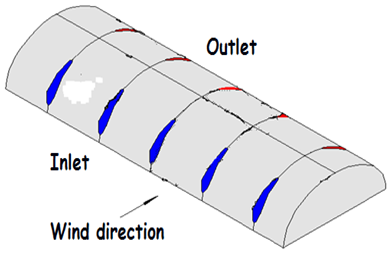(a)

## 1.2.png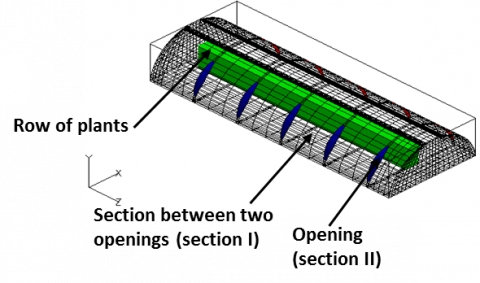(b)

Figure 1. Representation of the cultivated greenhouse equipped with openings. (a) Greenhouse geometry; (b) Mesh structure

At the inlet, wind speed was fixed at 2.3 m/s and the outside temperature has a value of 293 K.

The boundary conditions prescribed a null pressure gradient at the exit openings. The outlet air velocity is automatically calculated to satisfy the continuity equation.

The boundary conditions are summarized in Table 1.

Table 1. Boundary conditions for the studied greenhouse

 Boundary conditions Opening inlet Inlet: Velocity inlet: u=2.3ms-1 Temperature inlet: T=293K Opening outlet Pressure outlet Greenhouse cover Wall: Non-slip condition: u=v=w=0, q=15W.m-2 Greenhouse floor Wall: Non-slip condition: u=v=w=0, q=100W.m-2

The driving force of natural convection is the wind force and the buoyancy force arising from small temperature differences within the flow according to Bousinessq hypothesis.

4. Results and Discussion

Ventilation process affects significantly airflow and temperature distribution inside the greenhouses.

4.1 Airflow and temperature patterns under opened greenhouses without crop

Air circulation and temperature distribution are presented in Figures 2, 3 and 4.

In section located at the openings (section II), we observe that an important rate of flow penetrates through the windward opening, then a part of this flow crosses the tunnel according to the wind direction and a part flows in opposite direction creating a swirl near the exit opening.

## 1.png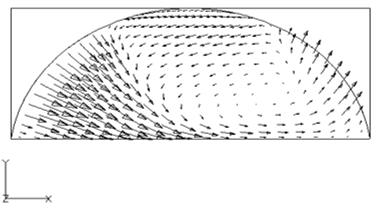(a)

## 2.png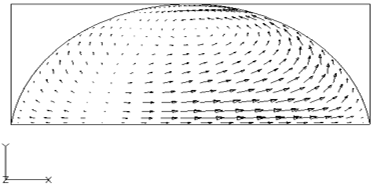(b)

Figure 2. Airflow patterns in the greenhouse without plant: (a) At section II; (b) At section I

## 3.1.png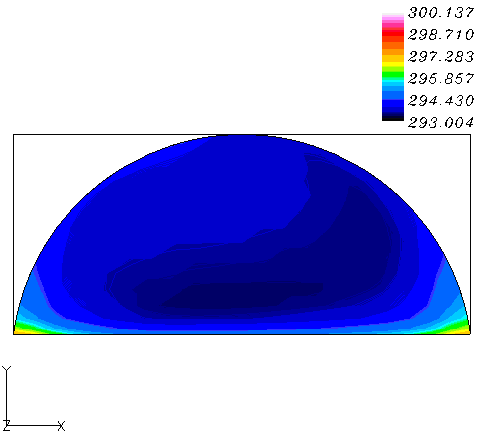(a)

## 3.2.png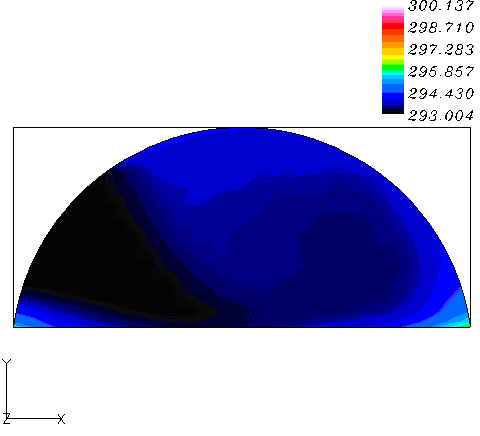(b)

Figure 3. Air temperature variation inside the greenhouse: (a) At section I; (b) At section II

In section I, located between the openings, the airflow is characterized approximately by a single rotating loop near the roof.

For, the calculated mean velocity, we observe that the velocity was lower in section I located between the openings ( $\bar{V}_{m o y}$ = 0.38 m/s) than that in section II located at the openings (Vmoy 1 m/s) by which air enters the greenhouse.

Air temperature patterns are given in Figure 3. In the majority of the inner volume, the temperature is constant except in the vicinity of the corners where the temperature variation exists. At windward opening, the temperature does not vary and its value is equal to the exterior one.  At section II, the greenhouse is colder than in section I because the air is better renewed in this region.

The field temperature near the floor is represented in Figure 4. This figure shows the heterogeneity of the temperature, it depends on the zones affected by the renewed air. So, this variation of temperature depends on position and section of the opening.

This variation in air velocity and temperature affects directly the development of the vegetation.

## 4.png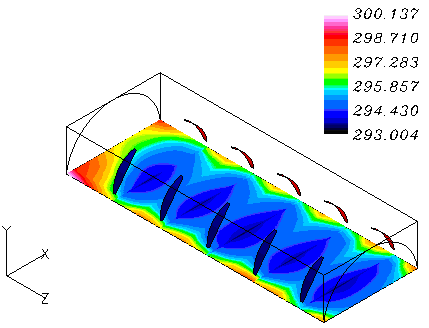Figure 4. Air temperature patterns near the floor of the opened tunnel greenhouse without crop

4.2 Airflow and temperature patterns under opened greenhouses with crop

The case of one row of plants occupying the center of the tunnel greenhouse is considered.

The greenhouse was heated and heating rate corresponds to the value of the solar radiation absorbed by the floor. Air circulation and temperature distribution are presented in Figures 5, 6 and 7.

The presence of the crop gives an irregular distribution of air movement in the greenhouse, with areas remaining unaffected by the air flow, and areas being under high air velocity. Air circulation has a direct effect on temperature variation and therefore it affects plant development. This was noticed by Fernandez and Bailey .

In section I, and due to the presence of the crop, airflow is characterized by two rotating loops (Figure 5 (a)), one is located in the center of the section and the other is located in the corner

In section II, we observe high-velocity along the windward opening and it has a value equal to that of the wind (Figure 5(b)). It is noticed, that the velocity decreases at the exit opening after deceleration of the air flow by the plant line and a part of the airflow flows in the opposite direction creating a loop.

In section I, the mean velocity is about $\bar{V}=33 \mathrm{cm} / \mathrm{s}$, it is lower than that in section II ($\bar{V}=86 \mathrm{cm} / \mathrm{s}$).

This variation in velocity affects the variation of the temperature and consequently, it influences the plant growth.

The temperature field in two sections is presented in the Figure 6.

The temperature has a heterogeneous variation inside the greenhouse. At the level of the opening, it is homogeneous and equal to the outside temperature, far from the openings and after the row of the plant, it is high because in this region, the air is less renewed.

Figure 7, represents a horizontal temperature distribution in the vicinity of the floor.

This figure shows clearly an increase in temperature in the non affected zones by the airflow. A symmetry is obtained in the temperature variation due to the position of the openings.

## 555555.png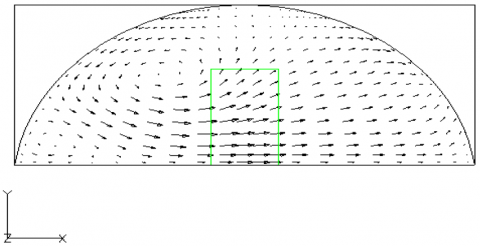(a)

## 6666666.png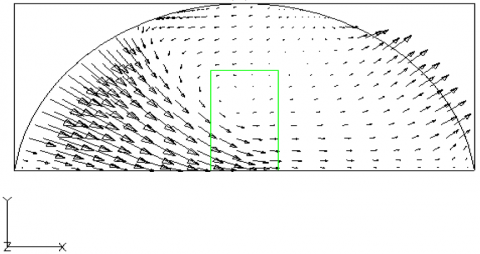(b)

Figure 5. Airflow patterns in the tunnel greenhouse with plants: (a) Section I, (b) Section II

## 6.1.png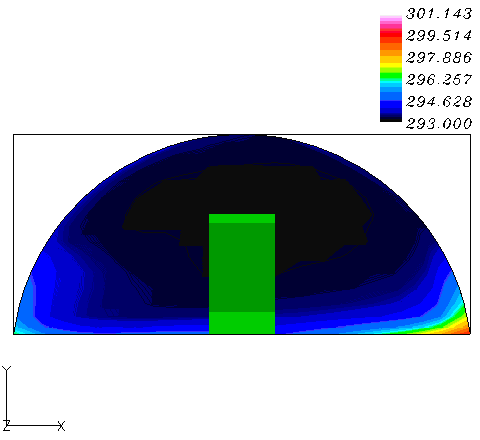(a)

## 6.2.png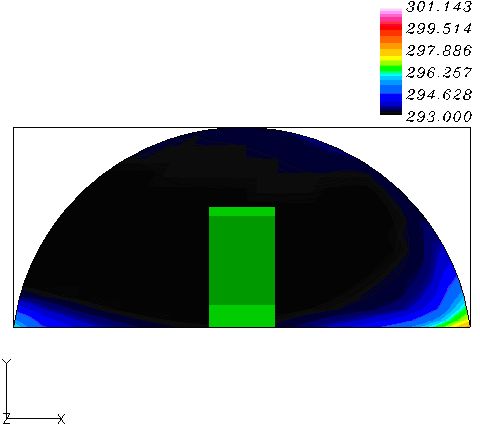(b)

Figure 6. Air temperature patterns in the opened tunnel greenhouse with crop: (a) Section I; (b) Section II

In this study, the buoyancy effect is the main driving force of the ventilation in the greenhouse. A cool stream of air entering the windward opening and the hotter air leaves through the leeward opening.

Air velocity inside the greenhouse had maximum values near the openings, whereas the air velocity was low in the middle of the greenhouse and at the plant region. The air velocity in the leeward opening was lower than that at windward opening.

In the greenhouse with crop, there was an elevated heterogeneity in the climate inside the greenhouse.

The temperature distribution shows the presence of temperature gradients close to the walls, this is due to the air movement. Another vertical gradient occurs above the surface of the floor which is due to the absorption of solar radiation.

Air entered the greenhouse with exterior temperature and left it warmer. Temperature variation and air circulation in the empty greenhouse are similar to those observed in the greenhouse with plant. The results obtained are satisfactory compared to those obtained in other similar studies [18, 19].

## 7.png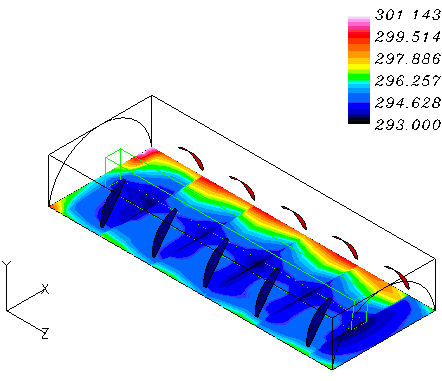Figure 7. Air temperature patterns near the floor of the opened tunnel greenhouse with crop

The results obtained show that position and section of the opening affects greenhouse micro-climate patterns and the spatial and temporal variations of the internal climate. So we can act on these two parameters in the regions where the air is less renewed. The side openings are more suitable for summer cooling, than the roof opening, which is confirmed by He et al. .

The rate of ventilation is not only the better indicator of ventilation performance but the temperature difference between crop and the air inside the greenhouse is also an indicator to take account.

5. Conclusions

In this study the influence of wind speed on the ventilation performance of tunnel greenhouse was investigated numerically by using a commercial fluid dynamics software Fluent-CDF based on the finite volume method. Airflows pattern and temperature distribution generated by a wind perpendicular to the openings of the tunnel greenhouse were numerically studied.

Small openings give reduction in velocity and increase temperature and the presence of the plant reduces airflow under greenhouse.

For this study, the value of the wind velocity was taken equal to 2.3 m/s. Small opening give reduction in velocity and increase temperature and the presence of the plant reduces airflow under greenhouse.

The presence of the crop gives an irregular distribution of the airflow in the greenhouse with regions with high air velocity and areas unaffected by the airflow.

The results produced by CFD compared well to other analytical results in literature in determining ventilation performance and three-dimensional calculations give more information on the ventilation processes.

Following the variation of the temperature, it is desirable to increase the section of the opening near the area where the air is less renewed or to see the possibility of placing more openings in the regions at low air circulation.

The number, the position and the opening area should be optimised to have a desired temperature for the growth of the plant, this can be done by calculating the difference in temperature between inside and outside. To have a more realistic description of the airflow and temperature distribution under greenhouse, mass exchanges between plant and air to be taken into account to know the plant influence on the microclimate under greenhouse.

The position and the opening areas should be optimised to have a desired temperature for the growth of the plant.

The computational fluid dynamics software Fluent-CDF is a power tool for the study of the greenhouse ventilation unlike simple laws used to determine the ventilation rate. The above results can give useful hints to improve the design and control the greenhouse ventilation.

Nomenclature
 k turbulent kinetic energy, m2 s-2 K permeability, m2 LAI crop leaf area index, p pressure, Pa Pr Prandtl number Re Reynolds number Sf source term T temperature, K To reference temperature, K u, v, w velocity components, m s-1 V velocity vector, m s-1 $\overline{\mathrm{V}}$ Mean Velocity, m s-1 x, y, z cartesian coordinates, m y+ Wall-normal distance, m Y inertial factor Greek symbols β volumetric expansion coefficient, K-1 µ dynamic viscosity, Kg m-1 s-1 ϕ physical quantity e viscous dissipation rate, m2 s-3 G diffusion coefficient, m2 s-1 r Density, Kg m-3 ro reference density, Kg m-3
References

 Bot, G.P.A. (1983). Greenhouse climate: From physical processes to a dynamic model. Ph.D Thesis, Agricultural University of Wageningen.

 Kanaan, M., Ghaddar, N., Ghali, K., Araj, G. (2015). Upper room UVGI effectiveness with dispersed pathogens at different droplet sizes in spaces conditioned by chilled ceiling and mixed displacement ventilation system. Building and Environment, 87: 117-128. http://dx.doi.org/10.1016/j.buildenv.2015.01.029

 Boulard, T., Baille, A. (1995). Modelling of air exchange rate in a greenhouse equipped with continuous roof vents. Journal of Agricultural Engineering Research, 61(1): 37-48. http://doi.org/10.1006/jaer.1995.1028

 Mistriotis, A., Bot, G.P.A., Picono, P., Scarascia-Mugnozza, G. (1997). Analysis of the efficiency of greenhouse ventilation using computational fluid dynamics. Agricultural and Forest Meteorology, 85(3-4): 217-228. http://doi.org/10.1016/S0168-1923(96)02400-8

 Kacira, M., Short, T.H., Stowell, R.R.A. (1998). CFD evaluation of naturally ventilated, multi-span, sawtooth greenhouses. Transactions of the ASAE, 41(3): 833-836. http://dx.doi.org/10.13031/2013.17222

 Ould Khaoua, S.A., Bournet, P.E., Migeon, C., Boulard, T., Chassériaux, G. (2006). Analysis of greenhouse ventilation efficiency based on computational fluid dynamics. Biosystems Engineering, 95(1): 83-98. http://doi.org/10.1016/j.biosystemseng.2006.05.004

 Okushima, L., Sase, S., Nara, M. (1989). A support system for natural ventilation design of greenhouse based on computational aerodynamics. Acta Horticulturae, 248: 129-136. http://dx.doi.org/10.17660/ActaHortic.1989.248.13

 Molina-Aiz, F.D., Valera, D.L., Alvarez, A.J. (2004). Measurement and simulation of climate inside Almeria-type greenhouse using computational fluid dynamics. Agric. For. Meteo, 125(1-2): 33-51. http://doi.org/10.1016/j.agrformet.2004.03.009

 Bournet, P.E., Boulard, T. (2010). Effect of ventilator configuration on the distributed climate of greenhouses: a review of experimental and CFD studies. Computers and Electronics in Agriculture, 74(2): 195-217. http://doi.org/10.1016/j.compag.2010.08.007

 Zeroual, S., Bougoul, S., Benmoussa, H. (2018). Effect of radiative heat transfer and boundary conditions on the airflow and temperature distribution inside a heated tunnel greenhouse. Journal of Applied Mechanics and Technical Physics, 59(6): 1008-1014. http://doi.org/10.1134/S0021894418060068

 Issa, R.I. (1985). Solution of the implicitly discretized fluid flow equations by operator-splitting. J. Comput. Phys, 62: 40-65. http://doi.org/10.1016/0021-9991(86)90099-9

 Patankar, S.V. (1980). Numerical Heat Transfer and Fluid Flow. McGraw-Hill. Washington, N.Y. http://dx.doi.org/10.1201/9781482234213

 Mohammadi, B., Pironneau, O. (1994). Analysis of the K-Epsilon Turbulence Model. Research in Applied Mathematics. Masson Wiley, New York.

 Forchheimer, P. (1901). Wasserbewegung durch boden. Z. Ver. Deutsch, 49: 1782-1788.

 Haxaire, R. (1999). Caractérisation et Modélisation des Ecoulements d’Air dans une serre. Thèse de Doctorat. Université de Nice Sophia Antipolis Nice, France.

 Chemel C. (2001). Modélisation et simulation des écoulements d’air dans les serres. Rapport de stage. Université Blaise Pascal, Clermont-Ferrand, France.

 Fernandez, J.E, Bailey, B.J. (1992). Measurement and prediction of greenhouse ventilation rates. Agricultural and Forest Meteorology, 58(3-4): 229-245. http://doi.org/10.1016/0168-1923(92)90063-A

 Boulard, T., Wang, S., Haxaire, R. (2000). Mean and turbulent air flows and microclimatic patterns in an empty greenhouse tunnel. Agricultural and Forest Meteorology, 100(2-3): 169-181. http://doi.org/10.1016/S0168-1923(99)00136-7

 Boulard, T., Wang, S. (2002). Experimental and numerical studies on the heterogeneity of crop transpiration in a plastic tunnel. Computers and Electronics in Agriculture, 34(1-3): 173-190. http://dx.doi.org/10.1016/S0168-1699(01)00186-7

 He, K.S., Chen, D.Y., Sun, L.J., Liu, Z.L., Huang, Z.Y. (2015). The effect of vent opening on the microclimate inside multi-span greenhouses during summer and winter seasons. Engineering Applications of Computational Fluid Mechanics, 9(1): 399-410. https://doi.org/10.1080/19942060.2015.1061553